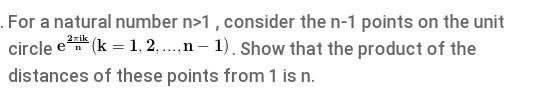# Complex Numbers

Viewing 2 posts - 1 through 2 (of 2 total)
• Author
Posts
• #70889#74433

The product of norms-norm of product

\prod_{k=1}^{n-1}||e^\frac}2\piik}{n}-1||=||\prod_{k=1}^{n-1}(e^\frac{2\piik}{n}-1)||

Let w_k=e^\frac{2\piik}{n} for roots of unity other than 1, these are the roots of the equation z^{n-1}+z^{n-2}+…+z+1=0

so,(w_k-1) is a root of

(z+1)^{n-1}+(z+1)^{n-2}+…+(z+1)+1=0 for all k.

The product of the roots of the polynomial are by Vieta’s formula equal to (-1)^{n-1}\frac{a_0}{a_n} where a_0 is the coefficient on the constant term a_n is the coefficient on the leading term

leading term’s coefficient is 1, the constant term is the sum of n 1’s.

product is (-1)^{n-1}n and its norm is n.

Viewing 2 posts - 1 through 2 (of 2 total)
• You must be logged in to reply to this topic.